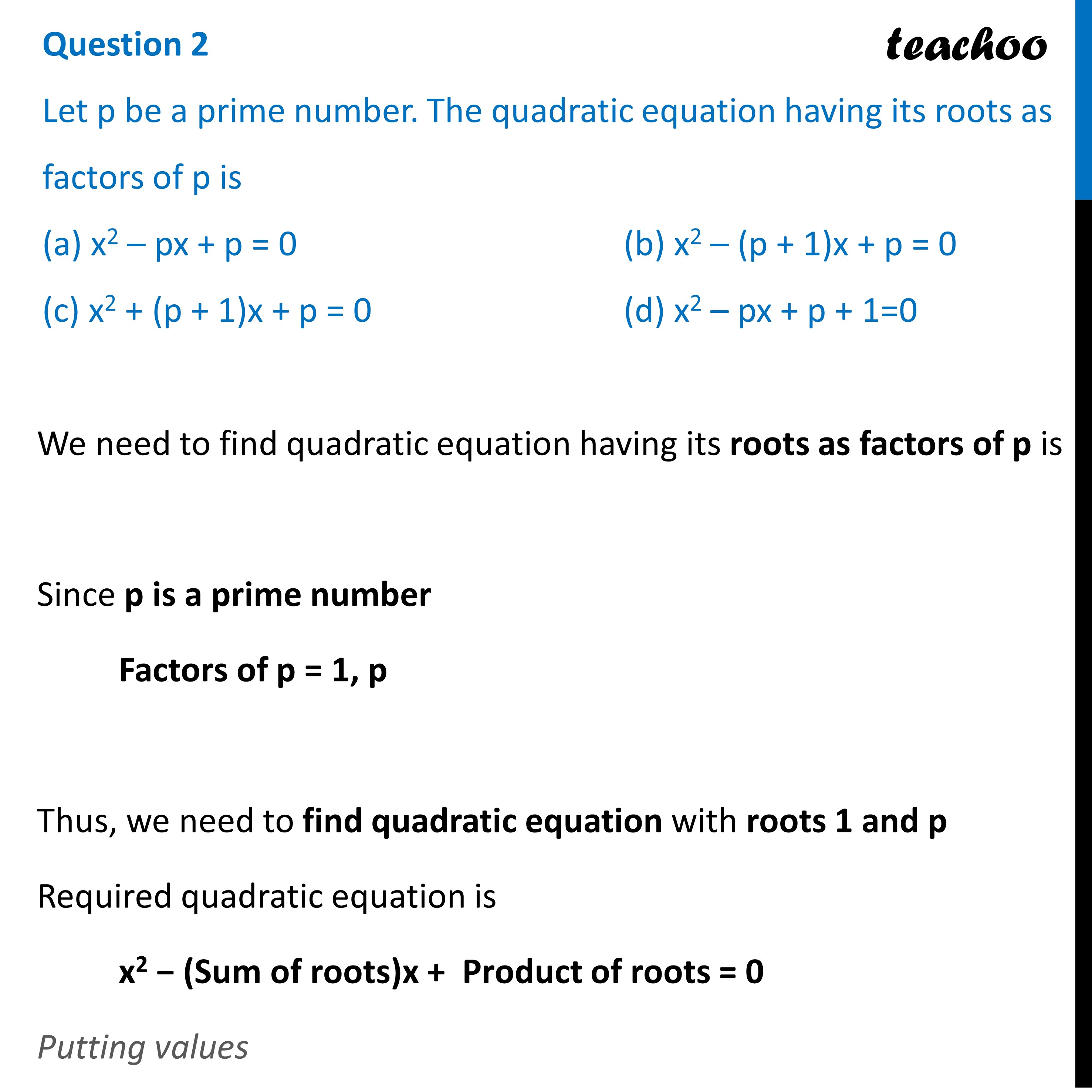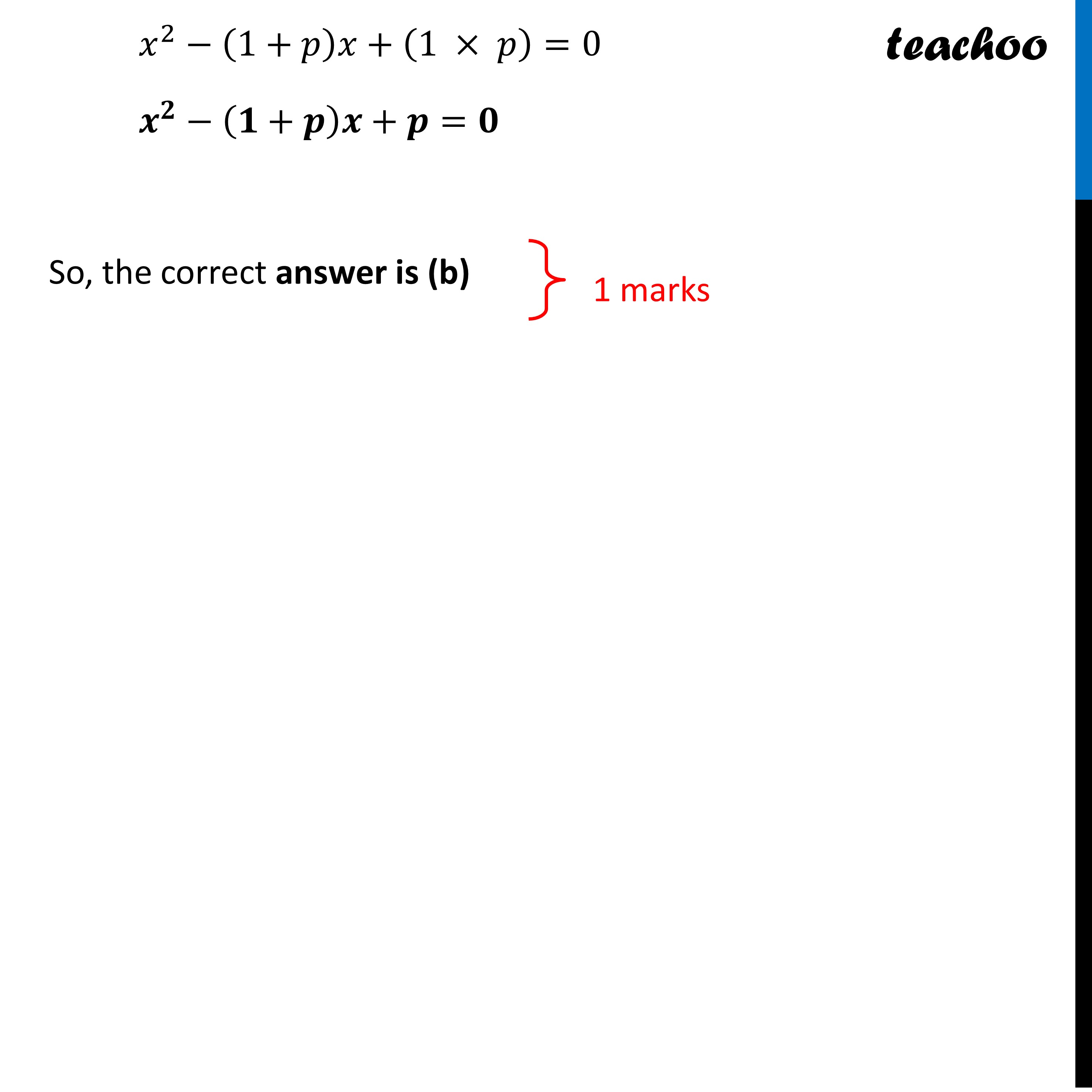CBSE Class 10 Sample Paper for 2023 Boards - Maths Standard

Class 10
Solutions of Sample Papers for Class 10 Boards

## (d) x 2 – px + p + 1=0Learn in your speed, with individual attention - Teachoo Maths 1-on-1 Class

### Transcript

Question 2 Let p be a prime number. The quadratic equation having its roots as factors of p is (a) x2 – px + p = 0 (b) x2 – (p + 1)x + p = 0 (c) x2 + (p + 1)x + p = 0 (d) x2 – px + p + 1=0We need to find quadratic equation having its roots as factors of p is Since p is a prime number Factors of p = 1, p Thus, we need to find quadratic equation with roots 1 and p Required quadratic equation is x2 − (Sum of roots)x + Product of roots = 0 Putting values 𝑥^2−(1+𝑝)𝑥+(1 × 𝑝)=0 𝒙^𝟐−(𝟏+𝒑)𝒙+𝒑=𝟎 So, the correct answer is (b)